Function Repository Resource:

# ContainsMissing

Test if an expression contains a Missing value

Contributed by: Arnoud Buzing
 ResourceFunction["ContainsMissing"][expr] gives True if expr contains Missing and False otherwise at level 1 of expr. ResourceFunction["ContainsMissing"][expr,lvl] gives True if expr contains Missing and False otherwise at level lvl of expr.

## Examples

### Basic Examples (2)

Check if an expression has a missing value at default level 1:

 In:=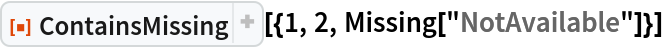Out=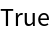Check if an expression contains a missing value (at default level 1):

 In:=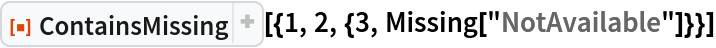Out=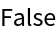At level 2, the expression contains a missing value:

 In:=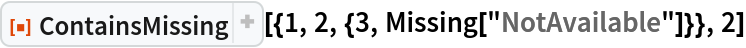Out=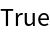### Scope (4)

Define some sample data with missing values:

 In:=Check if it contains missing values at the lowest level (-1):

 In:=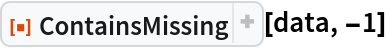Out=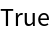Use with Select to get the element of the list with the missing value:

 In:=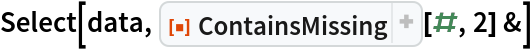Out=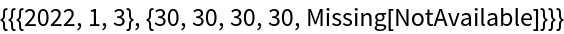Select the data that does not contain missing values:

 In:=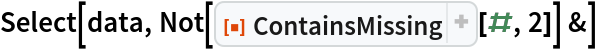Out=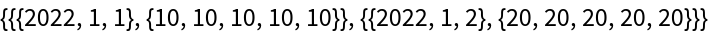### Possible Issues (1)

ContainsMissing does not yield True if only the symbol Missing is present; it yields True only if there is a Missing expression:

 In:=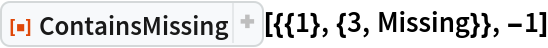Out=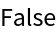In:=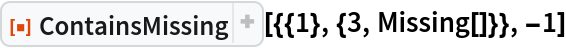Out=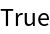Arnoud Buzing

## Version History

• 1.0.0 – 25 March 2022